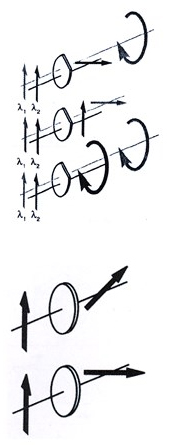## LENS TERMINOLOGY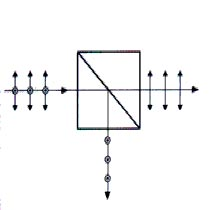The lens is the most basic optical component. It collects light from a source and refracts that light form a usable image of the source. The source may be an illuminated object or produce the light itself.

When designing experiments or assembling systems with simple lenses or lens combinations, there are a few formula that are very useful. The following definitions refer to the singlet lens diagram above. In the paraxial limits, however, any optical system can be reduced to the specification of the position of the principal and focal point. Shown on this diagram is the effective focal length (EFL or f), the back focal length (BFL), the front focal length (FFL), the center thickness(Tc), and the locations of the element’s principle points (H1 and H2). The radii of curvature R1 and R2 refer to the left and right surfaces respectively. R1 or R2 is positive(negative) if the center of curvature is to the right(left) side of the lens. The EFL is positive(negative) if the focal point is to the right(left).

## FOCAL LENGTH FORMULAS FOR SINGLET LENSES & MIRRORS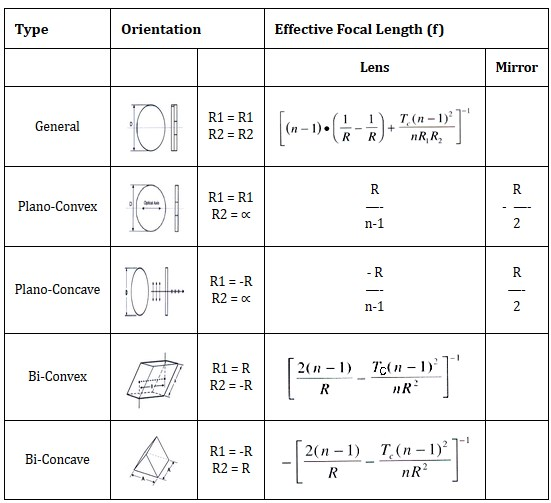where, n is index of refraction of lens material

## EFFECTIVE FOCAL LENGTH OF TWO THIN LENSES

The following formulas show how to calculate the effective focal length and principal point locations for a combination of any two thin lenses. Calculate the values for the first two elements, then perform the same calculation for this combination with next lenses. The expression for the combination focal length is the same whether lens separation distances are large or small and whether f1 and f2 are positive or negative.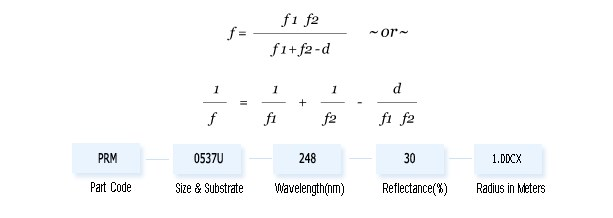## F-NUMBER (F/#)

The f-number (also known as speed) of a lens system is defined to be the effective focal length divided by system clear aperture(effective diameter D). The f-number defines the angle of the cone of light leaving the lens which ultimately forms the image. This is important concept when the throughput or light-gathering power of an optical system is critical, such as when focusing light into a monochromator or projecting a high power image.## NUMERICAL APERTURE (NA)

The numerical aperture of a lens system is defined to be the sine of cone angle,, that the marginal ray makes with the optical axis multiplied by the index of refraction(n) of the medium. The numerical aperture can be defined for any ray as the sine of the angle made by that ray with the optical axis multiplied by the index of refraction :## SAGITTA

The Sagitta (or Sag) of a spherical or cylindrical surface is an essential value to calculate when determining the edge and center thickness of a lens. The Sagitta is the thickness of material required to accommodate a surface of given radius of curvature with a given aperture. The Sag of surface may be calculated from :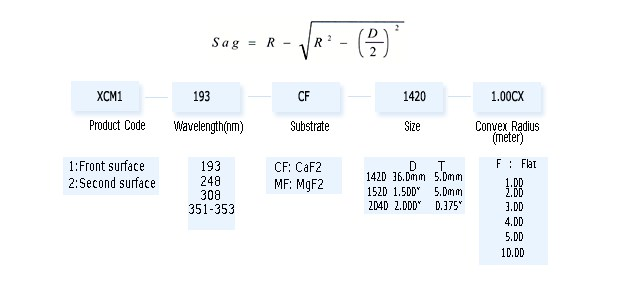## USING WEDGED WINDOWS

A problem with parallel substrate is that the second surface reflection can overlap the first surface reflection and lead unwanted spectral channeling through interference. The higher the parallelism, the worse the problem. Wedged windows are laser quality windows manufactured with wedges of 0.5Â° ~ 3Â°. For small angles of incidence, the deviation of ray incident on a wedged window with wedge angle α is 8 d = (n-1)α

The first reflected ray is misaligned from the initial axis by an angle defined by 8r = 2nα To create an adjustable beamsteering device, use a pair of identically wedged windows. Any deflection angle from 0 to 28, can be obtained by suitable rotation of the individual wedges. The direction of deflection in along a plane midway between the rotation angles of the two individual prisms. The magnitude of deflection varies smoothly from the maximum value, 2 where the thinnest points of the two wedges coincide to zero, when the two wedges are oriented antiparallel, thereby canceling each other.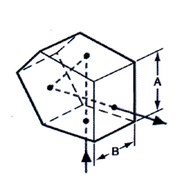Deviation and reflection of a beam by a wedged window of wedgeα.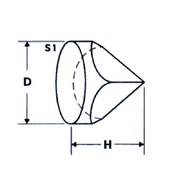A beamsteering wedge formed from two wedged prisms.

## SELECTING A PRISM

Prisms are blocks of optical material having flat polished sides arranged at precisely controlled angles to each other. Prisms may be used in an optical system to deflect or deviate a beam of light. They can invert or rotate an image, disperse light into its components wavelength, and be used to separate states of polarization. Prisms will introduce aberrations when used with convergent or divergent beam of light. Using prisms with collimated or nearly collimated light will help minimize aberrations.## WAVEPLATES

Waveplates operate by imparting unequal phase shifts to orthogonally polarized field components of an incident wave. This causes the conversion of one polarization state into another. These are two cases.

With linear birefringence, the index of refraction and hence phase shift differs for two orthogonally polarized linear polarization sates. This is the operation mode of standard waveplates.

With circular birefringence, the index of refraction and hence phase shift differs for left and right circularly polarized components. This is the operation mode of polarization rotator.

## QUARTER AND HALF WAVEPLATES

A quarter waveplate transforms polarized light between linear and circular polarization. Input linearly polarized light must be incident at 45Â° to the crystalline axis.

A half waveplate rotates the orientation of input polarized light. The rotation angle is twice the angle between the incident polarized light and the crystalline axis.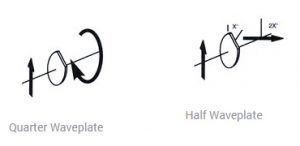## DUAL WAVELENGTH & HARMONIC WAVEPLATES

These are multiple order waveplates which provide specific retardance at two different wavelengths (ex. quarter wave at 1064nm and half wave at 532nm). Dual wavelength waveplates are offered at a variety of popular laser wavelengths and harmonic waveplates are used at 1064nm and various harmonics.

Dual Wavelength waveplates provide separation of different wavelengths with a polarization beamsplitters by rotating the polarization of one wavelength by 90Â°.

These components are particularly useful when used in conjunction with other polarization sensitive components to separate coaxial laser beams of different wavelength.

## POLARIZATION ROTATORS

The optical axis in a polarization rotator is perpendicular to the polished face of the optic. The result is that the orientation of input linearly polarized light is rotated as it propagates through the device. Standard devices offer 45Â° and 90Â° rotation at a number of common laser wavelengths. Unlike a half waveplate, the rotation is invariant to the polarization of the incident light.

The polarization rotators is highly dependent (nearly inverse square ratio) upon wavelength.